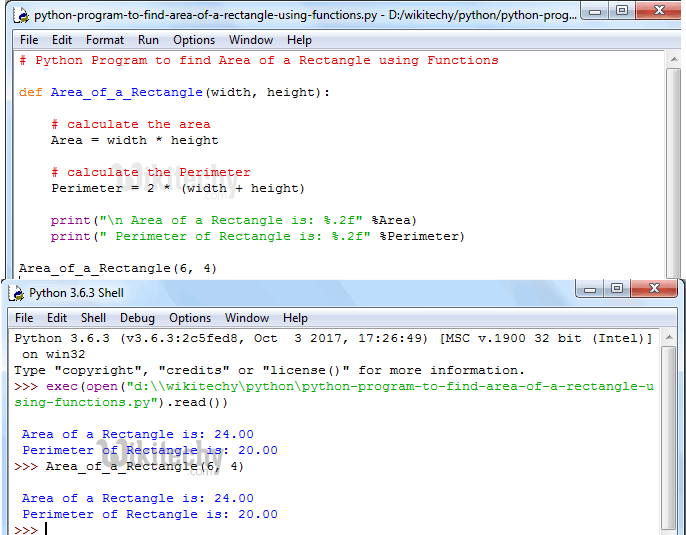# python tutorial - Python Program to find Area of a Rectangle - learn python - python programming

• To write Python Program to find Area of a Rectangle and Perimeter of a Rectangle with example.
• Before we step into the program, Let see the definitions and formulas behind Perimeter and Area Of a Rectangle.Learn Python - Python tutorial - Python Program to find Area of a Rectangle - Python examples - Python programs

## Area of a Rectangle

• If we know the width and height then, we can calculate the area of a rectangle using below formula.
• Perimeter is the distance around the edges. We can calculate perimeter of a rectangle using below formula

## Python Program to find Area of a Rectangle and Perimeter of a Rectangle

• This program allows the user to enter width and height of the rectangle. Using those values we will calculate the Area of a rectangle and perimeter of a rectangle.

## OutputLearn Python - Python tutorial - Python Program to find Area of a Rectangle and Perimeter of a Rectangle - Python examples - Python programs

## Analysis

• Following statements will allow the User to enter the Width and Height of a rectangle.
• Next, we are calculating the area as per the formula
• In the next line, We are calculating the Perimeter of a rectangle
• Following print statements will help us to print the Perimeter and Area of a rectangle

## Python Program to find Area of a Rectangle using functions

• This program allows the user to enter the width and height of a rectangle.
• We will pass those values to the function arguments to calculate the area of a rectangle.

## Analysis

• First, We defined the function with two arguments using def keyword.
• It means, User will enter the width and height of a rectangle.
• Next, We are Calculating the perimeter and Area of a rectangle as we described in our first example.

## OutputLearn Python - Python tutorial - Python Program to find Area of a Rectangle using functions - Python examples - Python programs

NOTE: We can call the function with arguments in .py file directly or else we can call it from the python shell. Please don’t forget the function arguments